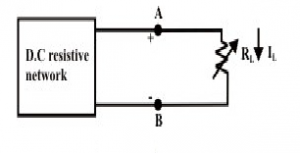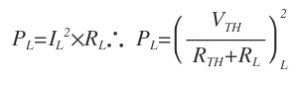Home > dccircuits > maximum power transfer theorem

# Maximum Power Transfer Theorem

Statement of maximum power transfer theorem :
The maximum power transfer theorem states that the maximum amount of power will be delivered to the load resistance when the load resistance is equal to the Thevenin /Norton resistance of the network supplying the power. If the load resistance is lower or higher than the Thevenin /Norton resistance of the source network, then the power delivered to load is less than maximum.
That means the condition for maximum power transfer according to maximum power transfer theorem is, RL = RTH
General statement :
In an active network, maximum power will be transferred from source to load if the load impedance is the complex conjugate of the internal impedance of the circuit as seen from terminals of the load.

In an electric circuit, the load receives electric energy via the supply sources and converts that energy into a useful form. The maximum allowable power receives by the load is always limited either by the heating effect (in case of resistive load) or by the other power conversion taking place in the load. The Thevenin and Norton models imply that the internal circuits within the source will necessarily dissipate some of power generated by the source. A logical question will arise in mind, how much power can be transferred to the load from the source under the most practical conditions? In other words, what is the value of load resistance that will absorbs the maximum power from the source? This is an important issue in many practical problems and it is discussed with a suitable example.Let us consider an electric network as shown in above figure, the problem is to find the choice of the resistance RL so that the network delivers maximum power to the load or in other words what value of load resistance RL will absorb the maximum amount of power from the network. This problem can be solved using nodal or mesh current analysis to obtain an expression for the power absorbed by RL, then the derivative of this expression with respect to RL will establish the condition under what circumstances the maximum power transfer occurs. The effort required for such an approach can be quite tedious and complex. Fortunately, the network shown in figure can be represented by an equivalent Thevenin's voltage source as shown in below figure.

In above figure a variable load resistance RL is connected to an equivalent Thevenin circuit of original circuit. The current for any value of load resistance is,$I_{ L } = \frac { V_{ TH } }{ R_{ TH }+R_{ L } }$

Then, the power delivered to the load is..MaximumPowerTransferTheorem

The load power depends on both; however, is constant for the equivalent Thevenin network. So power delivered by the equivalent Thevenin network to the load resistor RL is entirely depends on the value of RL. To find the value of RL that absorbs a maximum power from the Thevenin circuit, we differentiate PL with respect to RL
For maximum power dissipation in the load, the condition given below must be satisfied$\frac { d^{ 2 }P(R_{ L }) }{ d{ R_{ L } }^{ 2 } } =\frac { { V_{ TH } }^{ 2 } }{ 8R_{ TH } } <0$

This result is known as â€œMatching the loadâ€ or maximum power transfer occurs when the load resistance RL matches the Thevenin's resistance RTH of a given systems. Also, notice that under the condition of maximum power transfer, the load voltage is, by voltage division, one-half of the Thevenin voltage.

The expression for maximum power dissipated to the load resistance is given by,$P_{ max }={ \left( \frac { V_{ TH } }{ R_{ TH }+R_{ L } } \right) }^{ 2 }\times R_{ L }\\ \therefore \quad P_{ max }=\frac { { V_{ TH } }^{ 2 } }{ 4R_{ TH } }$

The total power delivered by the source is$P_{ r } = { I_{ L } }^{ 2 }(R_{ TH }+R_{ L }) = 2\times { I_{ L } }^{ 2 }R_{ L }$
This means that the Thevenin voltage source itself dissipates as much power in its internal resistance RTH as the power absorbed by the load RL.

Efficiency under maximum power transfer condition is given by$efficiency = { \frac { { I_{ L } }^{ 2 }R_{ L } }{ 2\times { I_{ L } }^{ 2 }R_{ L } } \times 100 } = 50%$